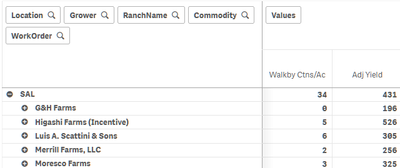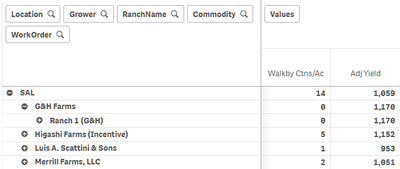App Development

Announcements
QlikWorld 2022, LIVE in Denver CO., May 16-19, 2022. REGISTER NOW TO RECEIVE EARLY BIRD PRICING
cancel
Showing results for
Did you mean:Creator III

Sum formula is not working correctly in pivot table

I have the following formula for Adjusted Yield in my pivot table:

If(Sum(WalkbyQty) > 0, (Sum(EU_RecQty) / Sum(Acres)) + (Sum(WalkbyQty) / Sum(Acres)),(Sum(EU_RecQty) / Sum(Acres)))

In my rows, I have five dimensions for drill down.  The Adjusted Yield formula does not calculate correctly at the top two levels; however, if I drill down further, then the formula appears to work correctly.  I have a feeling this formula might require more refinement such as with the Aggr function, but I would like to get some feedback and suggestions please.  Thank you.

Example of incorrect calculation for Adj Yield:Drill down one level on G&H Farms and all numbers suddenly are calculated correctly:12 RepliesCreator III
Author

I haven't been able to modify your formula to work correctly at all levels.  Is there another approach I should consider?MVP

@mikegrattan  Your data model looks complex and this is the reason why you are facing this issue. Issue is that you have null values for Commodity dimensions, so when you drill down to to lower level pivot does not show null values and calculates correctly. At higher level, sum function always sums entire set of values. To avoid this one workaround is below.

If(Sum({<Commodity={"*"}>}WalkbyQty) > 0, (Sum({<Commodity={"*"}>}EU_RecQty) / Sum({<Commodity={"*"}>}Acres)) +
(Sum({<Commodity={"*"}>}WalkbyQty) / Sum({<Commodity={"*"}>}Acres)),
(Sum({<Commodity={"*"}>}EU_RecQty) / Sum({<Commodity={"*"}>}Acres)))

I am not sure if this completely solve the issue, but if at other level null values comes up and you don't want to consider that then you may also need to include the condition for that dimension like eg.

Sum({<Commodity={'*'}, Rower={'*'}>}Acres)Creator III
Author

@Kushal_Chawda Yes, that helps to explain what's going on.  Some of the data being loaded doesn't include Commodity, so that could leave null values in certain analyses.  I will try your suggestions.  Thank you.Tags
Community Browser